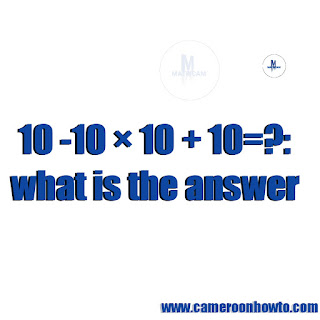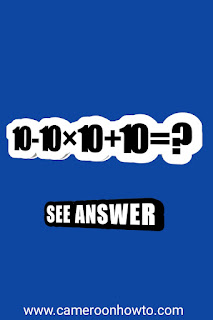1

# 10 -10 × 10 + 10=?: what is the answer

The solution to What is 10 -10 × 10 + 10=? Answer? simple but tricky. Many people always fall into the trap of not using basic mathematical principles and rules such as BODMAS and PEMDAS . BODMAS stands for: B=Bracket, O=Order of operation, D=Division, M=Multiplication, A=Addition, S=Subtraction while PEMDAS stands for P=Parentheses, E=Exponents, M=Multiplication, A=Addition and S=Subtraction.# 10 -10 × 10 + 10=?: what is the answer

So solving this question using BODMAS, we take one step and explain  before going to another for better understanding.

This question has gone viral on social media and many people has answers as 10 and -80. If you have been doubting your answer, then in this article I will solve and explain  in details why the answer is -80 Taking into consideration some Basic Mathematical rules such as :

TRENDING MATH QUIZ:
If A + A = 30, B+B = 20, C+C = 8, What's A+B ×C= ? answer
• Order of Operation (BODMAS and PEMDAS).
• Solving from left to right.
• Signs lies behind a number. This rules is affecting many answers so you have to take it serious and master your number line well.school supplies

## ANSWER TO 10 -10 × 10 + 10=?

Firstly I start we multiplication, that is

-10×10=-100.

Remember negative is attached to the second 10. So now we have

10-100+10. Note that negative affects 100 here not 10 because in arithmetic, signs such as +×÷- are attached to number from the left not right.

Secondly we go straight to addition since there is no division sign in the question. If we arrange the work in order of BODMAS, it will be as thus:

10+10-100=?
20-100=?
-80=?

##Summary answer to 10-10×10+10=?

In summary, the answer to 10-10×10+10=? is -80 see solving below

10-10×10+10=?
10-100+10=?
10+10-100=?
20-100=?

-80=?.

## Check more similar examples below.

1+1-1+1=?1+1-1+1=?

Solution:

If we apply BODMAS here, the answer will be as follows.

1+1-1+1=?

1+1+1-1=?

3-1=?

2=?

2-2×2+2=?2-2×2+2=?

Solution:

2-2×2+2=?

2+2-2×2=

4-4=?

0=?.

If we always solve our arithmetic questions using the rules above, our answers will always be the same and if not then mathematics would have been the most complicated subject on earth.

From the main quiz above, ,many people have 20 due to wrong application of BODMAS.

I hope you learn some tricks in math today.

Now comment your views or share with friends. Learning never ends!

Tags

1.